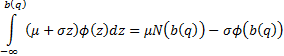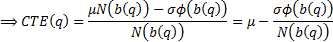/

### Identifying a formula for the (lower) conditional tail expectation (CTE) of a normal distribution that does not explicitly include integral signs but instead refers to the unit normal density function and the unit normal cumulative distribution function

The (lower) conditional tail expectation (CTE) of a random variable for a given cut-off,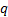, is defined as the expected value of the random given that it is below the relevant cut-off. It is thus very closely aligned with the Tail Value-at-Risk (TVaR) of the distribution, since the TVaR is the CTE for a specific cut-off. For a normal distribution the CTE is thus: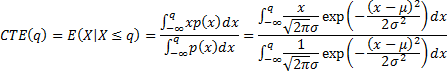The unit normal distribution function,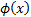, and the unit Normal cumulative distribution function,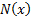, are defined as follows: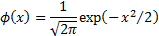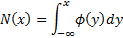Substituting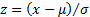in the above formula for the CTE we obtain, where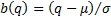):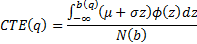We can re-express this in a form that relies only onandby noting the following: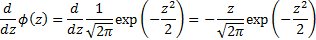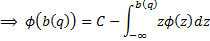where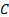is constant and furthermore as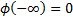and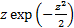decays sufficiently fast to zero as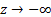we have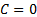. Hence: